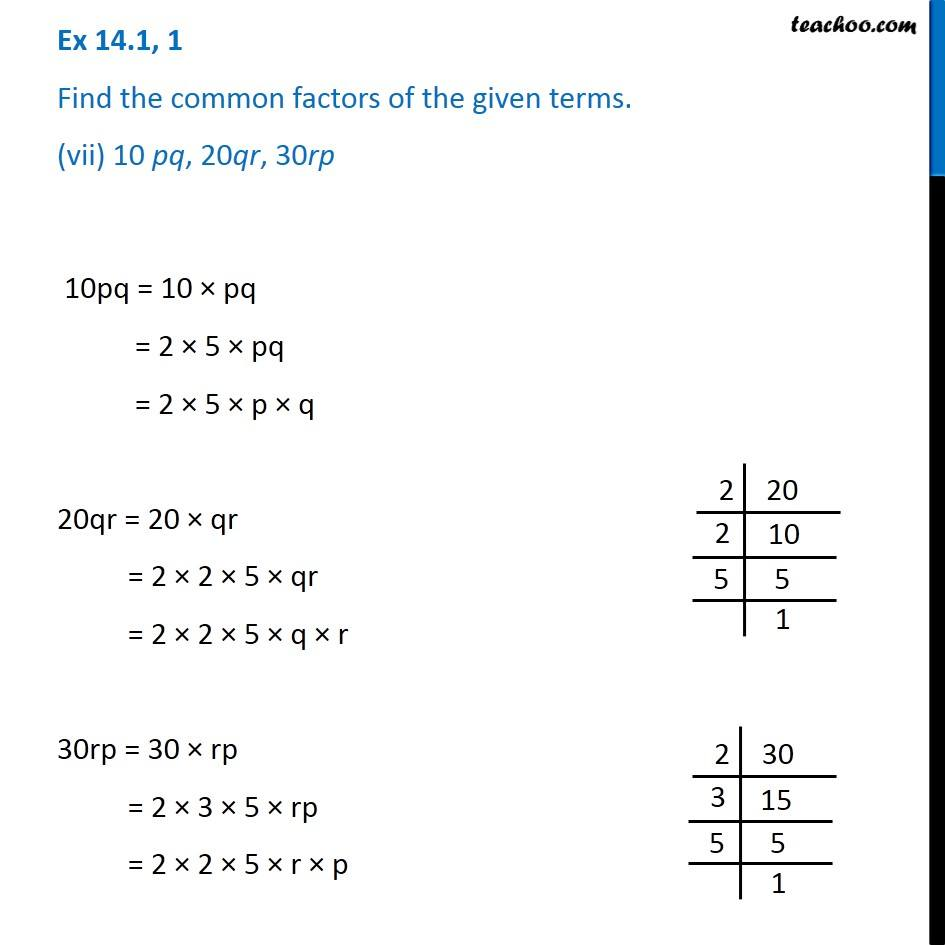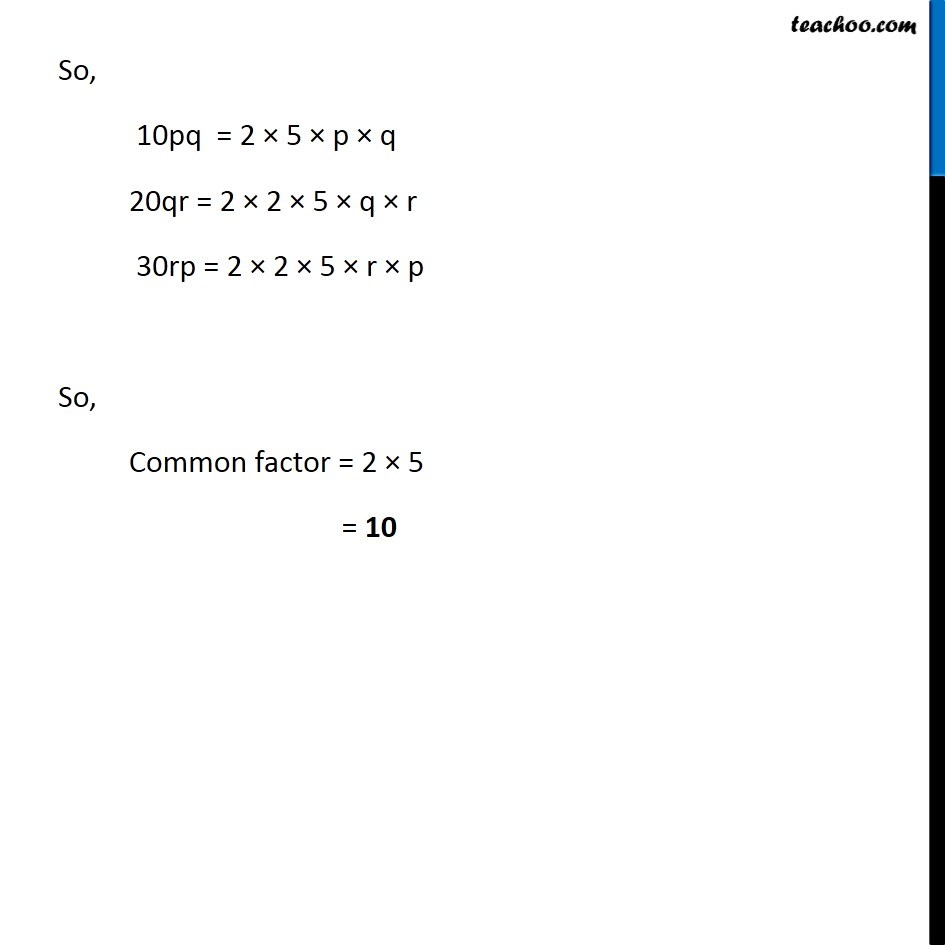1. Chapter 14 Class 8 Factorisation
2. Serial order wise
3. Ex 14.1

Transcript

Ex 14.1, 1 Find the common factors of the given terms. (vii) 10 pq, 20qr, 30rp 10pq = 10 × pq = 2 × 5 × pq = 2 × 5 × p × q 20qr = 20 × qr = 2 × 2 × 5 × qr = 2 × 2 × 5 × q × r 30rp = 30 × rp = 2 × 3 × 5 × rp = 2 × 2 × 5 × r × p So, 10pq = 2 × 5 × p × q 20qr = 2 × 2 × 5 × q × r 30rp = 2 × 2 × 5 × r × p So, Common factor = 2 × 5 = 10